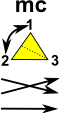# Maths - Equilateral Triangle Transforms

## Dihedral Group D3

In this example lets take the mapping of the equilateral triangle to itself, that we looked at in the introduction, but this time we will also include reflections. So we end up with the identity, 2 possible rotations and 3 possible reflections. This is all the possible permutations so this is a symmetry group:

  permutation cycle notation identity (0°) anticlockwise (120°) clockwise (240°)(1)(2)(3) (1 2 3) (3 2 1) reflect in 1 - 23 reflect in 2 - 13 reflect in 3 -12(1)(2 3) (2)(1 3) (3)(1 2)

So, what is the minimum set of generators to generate this group?

We can generate the rotations by combining two reflections:====So the 3 reflections will generate the group, but we don't need 3 because once we have one rotation we can generate the other reflection.

So 2 permutations are needed to generate the whole group, we can either use:

• 2 different reflections, or
• 1 reflection and 1 rotation

however we can't use 2 rotations because rotations form a self contained subset of this group.

So, as an example, lets choose the generators to be 'a' and 'b' where:

• a = ma reflection through in 1 - 23
• b = ma reflection through in 2 - 13

We can generate all the permutations as follows:

• e = 1
• ma = a
• mb = b
• mc = aba
• ra = ba
• rb = ab

## Cayley Table

The complete table is:

 1 a b aba ba ab 1abababaabor we can show this completely in terms of the generators:

 1 a b aba ba ab 1 1 a b aba ba ab a a 1 ab ba aba b b b ba 1 ab a aba aba aba ab ba 1 b a ba ba b aba a ab 1 ab ab aba a b 1 ba

## Ways to define the structure of this group

What is the minimum information required to define the structure of this group? We could:

• Specify each permutation in the group by using the 3 arrows as shown above for each permutation.
• Specify each permutation in the group by using the cycle notation for each permutation.
• By the Cayley table for the group.

Another way to define the group is the 'Presentation of the group', as explained on this page, for this example it is:

Γ< a,b | aa,bb,bab=aba>

We could have set all relations to be equal to unity by replacing bab=aba with baba-1b-1a-1=1

Can we use this to generate all the entries in the table and thus competely define the structure?

Lets take an example of multiplying 'ab' by 'ab':

ab * ab = abab [associativity]

= aaba [since bab=aba]

= ba [since aa=1]

Doing this for all the other entries in the Cayley table shows that this competely defines the group. How can we work out the minimum set of relations that will define the group?

## Cayley Graph

Upto now we have chosen to use two reflections for the generators but it is more common to use 1 rotation and 1 reflection because can be more easily generalised to an 'n' sided dihedral. This is given by one cycle going clockwise and another going anticlockwise connected by the reflections.

Here are Cayley graphs for the case where the generator is 1 rotation + 1 reflection and the case where the generator is 2 reflections.

Generator 2 reflections 1 rotation + 1 reflection
Presentation < a,b | aa,bb,bab=aba> < a,b | aa,bbb,a-1ba=b-1>
GraphGiven two Cayley graphs can we find if they represent the same group?

## Sandwich Product

The form y x y-1 has some interesting effects:

For instance if y is a rotation and x is a reflection, then we get a different reflection=Or if x is a rotation and y is a reflection, then we get the inverse rotation.=So: m r m -1 = r -1

To find out more about this subject search for 'Combinatorial Group Theory' (don't confuse with combinatorics which is a different branch of mathematics).

Book Shop - Further reading.

Where I can, I have put links to Amazon for books that are relevant to the subject, click on the appropriate country flag to get more details of the book or to buy it from them.The Princeton Companion to Mathematics - This is a big book that attempts to give a wide overview of the whole of mathematics, inevitably there are many things missing, but it gives a good insight into the history, concepts, branches, theorems and wider perspective of mathematics. It is well written and, if you are interested in maths, this is the type of book where you can open a page at random and find something interesting to read. To some extent it can be used as a reference book, although it doesn't have tables of formula for trig functions and so on, but where it is most useful is when you want to read about various topics to find out which topics are interesting and relevant to you.